Select Page

On L’ag B’Omer this year I learned of a powerful Divine sequence of 7 numbers: 22, 23, 26, 32, 42, 57, and 78.  These are all spiritual numbers with powerful connections to Names of G-d individually in their own right. The other thing I learned that night was the importance of the irreducible prime numbers within G-d’s divine plans. I apologize for all those whose eyes glaze over at the sight of numbers, but we cannot yet glimpse into Heaven and have only its shadows upon our world, which are reflected through the Hebrew letters and their associated numbers (their energetic equivalents).
The number 22 represents the 22 letters of the Hebrew Alphabet.The number 23 represents the ordinal value of the angel code (יאל) written into most of their names, including Israel (ישראל). It is also the ordinal value of every other syllable in the all-important first verse of the Torah from the כל of Kol Israel (the last 2 words of the Torah) to the ברא of Bereshit and of Bara, the ים of Elohim and of H’Shamayim, and the את of Et and of V’Et.  It is also a connection to healing through the 23 verses of the Shema prayer, the 23 words of the blessing of the Cohenim, and the 23 pairs of chromosomes in our bodies.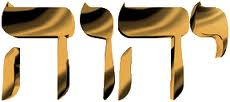The number 26 represents the Tetragrammaton (יהוה).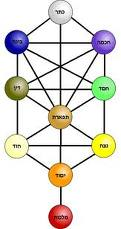The number 32 represents the 32 Paths of Wisdom of the 10 sefirot of the Tree-of-life. By the way, 23 is the 10th Prime number.

The number 42 is representative of the 42-Letter Name of G-d. Finally the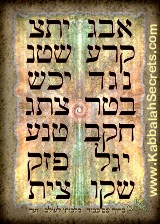numbers 57 and 78 are part and parcel to the year 5778 and built into the 42-Letter Name through its 5th triplet (נגד), whose value and complete value, respectively, are 57 and 78. The number 78 is also kabbalistically noted as 3 times the value of the Tetragrammaton (יהוה).

57 and 78 or 5778 are also reflected in the 72 Names of G-d matrix through the placement of the only double crowned triplets in it: (ננא) and (ענו) at columns 5, row 7, and column 7, row 8 respectively.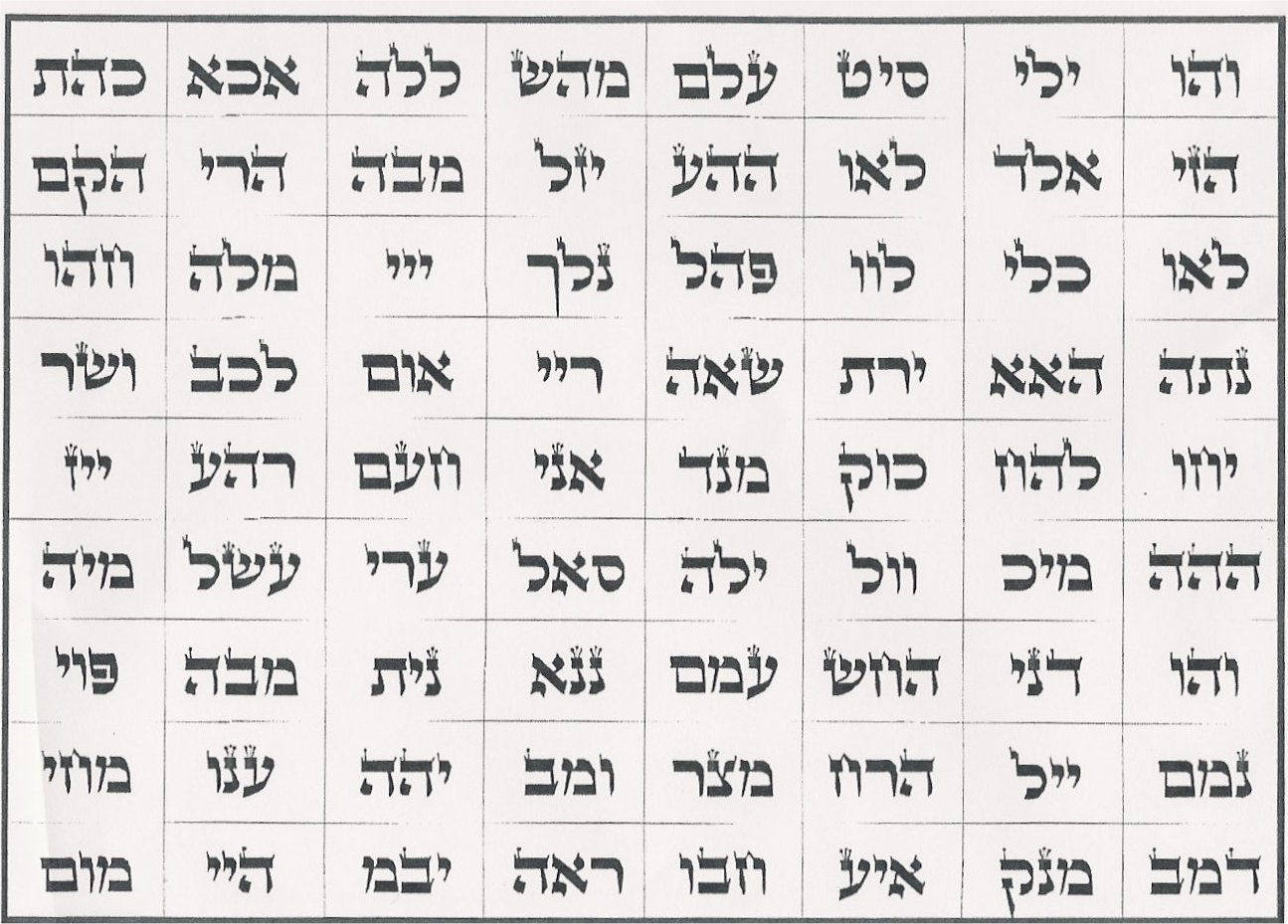Curiously, the triplet (ננא) has the numerical value 101, which is the sum of the prime numbers from 1 to 23.  Even more curious, the number 101 is the 26th prime number, which is notable because 101 is the numerical value of Michael, the guardian Archangel of Israel.  Moreover, the sum of all the Hebrew letters through the 23rd Hebrew letter, the final Caf (ך) plus the kolel for the 23 letters is 2018, matching the year 5778 in the Hebrew Calendar.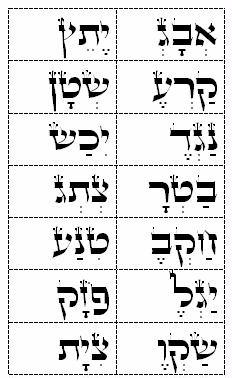These select set of Divine numbers also interact with each other, as in 22 x 23 equals 506, the complete value of “Moshiach Ben David” and the value of the first line of the 42-Letter Name and the 506 occurrences of the 14 triplets of the 42-Letter Name in the Torah, etc.  Another example is 26 x 32 equaling 832, as in the 832 years the two Holy Temples stood (422 and 410 years respectively), representing 1/4 of the time period between the giving of the Torah at Sinai in 2448 HC and Shavuot 5778.  832 years is also the time frame from the construction of the First Holy Temple in 2928 HC to 3760 HC, the pivotal year “0” chosen for the Gregorian calendar, and it is the number of years from the Flood to Moses’ death in 2488.
All that be as it may, the 7 numbers (22, 23, 26, 32, 42, 57, and 78) form a sequence, one built repeatedly into the Torah as well.  The sequential difference between these numbers (23-22, 26-23, 32-26…) is 1, 3, 6, 10, 15, and 21, which is the familiar triangular number sequence often used in the Torah.  It represents the sum of integers up to the numbers 1, 2, 3, 4, 5, and 6 respectively (i.e. 1, 1+2, 1+2+3…).  One example is that the first verse of the Torah is comprised of 7 words, alternatively 28 letters, and the sum of the integers from 1-7 is 28.  Moreover the sum of the gematria value of those 28 letters is equivalent to the sum of the integers from 1 -73, so it is no coincidence that 28 +73 = 101.
Another example is that all the occurrences in the Torah of all the Yuds(י), Heis (ה) and Vavs (ו) in the Torah, the components of the Tetragrammaton (יהוה) is exactly 90,100, which is the sum of the integers from 1  – 424, the numerical value of Moshiach Ben David.  Yet another example is found in the prophesied date 5778, which is the sum of the integers from 1 – 107. So it is further noteworthy that the sum of Yud(י), Hei (ה) and Vav (ו) is 21 and the 21st prime number is 73, connecting those 90,100 back to the Torah’s first verse and Chochma (wisdom) of numerical value 73.  Yet another connection back to that most powerful verse from which the 42 Letter Name emanates  is that the 28th prime is 107. By the way, 28 + 107 = 135, which is the same as 57 and 78.
Numbers represent energy and energy is never lost, just concealed.  Therefore, in essence, this divine sequence (22, 23, 26, 32, 42, 57, and 78) represents cumulatively the combined energy of the numbers 1 through 6 added to the 22 letters of the Hebrew Alphabet.  These 6 cumulative energies (1, 3, 6, 10, 15, and 21), represented by the Hebrew letters (א,ג,ו,י,ס,ש) respectively have the complete gematria value of 424 when the koleel of 6 is subtracted.  Likewise, when we multiple the numerical value of the Yud (י), Hei (ה) and Vav (ו), we get 300 and when we spread the light by squaring as instructed by R’Chaim Vittal of blessed memory, we get 90,000. Then adding the 100, representing Keter for completion, and as in the 100th prime number, 541, the value of Israel (ישראל), we get 90,100.  Moreover the squares of the first two letters in Israel (ישראל) also equal 90,100, while the last two equal 901.
Right in the middle of the Torah’s first verse is the article ET (את), which as noted above has an ordinal value of 23. It has a complete value of (401 + 23) or 424, Moshiach Bed David once again.
Suffice to say, G-d has a plan. G-d designed the Torah to bring us Moshiach Ben David in the right moment and He gave us these powerful Names of His to help connect us daily to that energy.While completely unexpected, it should be no surprise that those sequential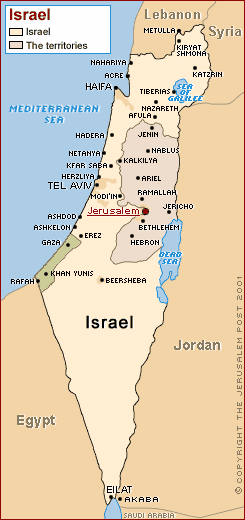Names (22, 23, 26, 32, 42, 57, and 78) sum to 280, as in the sum of the 5 powerful final letters (ךםןףץ) in the Hebrew alphabet, the ones associated with severe judgment and the end of days.  This is no mathematical slight of hand. The Names and numbers are G-d given. Abraham first wrote about the 22 building blocks and their values some 3760 years ago.  And the fact that the first and last numbers (22 and 78) add up to 100, like the 100th prime, 541 (Israel), is the same as the middle 3 Divine numbers (26, 32 and 42) cannot be made up or manipulated.  They are simply what was given to us.  Furthermore, the sequential spacing between those Names numbers (1, 3, 6, 10, 15, 21) sum to 56, just like the sum of the two middle numbers in the sequence (26 and 32) with 5 times 56 being 280 as well.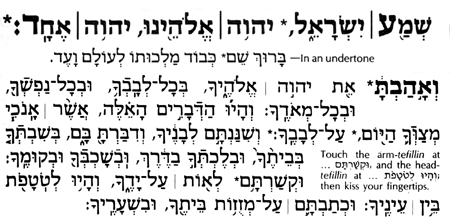We have advised repeatedly over the years that beside doing the mitzvot with the proper kavannot and consciousness the best, highest, and easiest way to connect to this energy of Moshiach and the sea of Binah (the World to Come) is through the Torah’s first verse, the 42-Letter Name of G-d and the Shema prayer, so it should not be a surprise to us that when we add the prime numbers that are associated with the 7 Divine Name sequence (79, 83, 101, 131, 181, 269 and 397) they sum to 1248 including the kolel of 7, which is equivalent to the sum of the 1000 letters and 248 words in the Shema Prayer that come directly from 3 specific paragraphs in the Torah.
Nor is it a surprise that the middle 3rd and 4th primes of this sequence sum to 232, as in the full 4 aspects of the Tetragrammaton (יהוה), or that the final two primes, which sum to 666, as in 66.6 jubilee years that stretch from the first Giving of the Torah at Sinai and this Shavuot in 5778.  The three other primes (79, 83, and 181), corresponding to 22, 23 and 42 of the divine sequence, sum to 343.  This is also the sum of the location and value of the two “57-78” triplets from the 72 Names, the 53rd one (ננא) of value 101 and the 63rd one (ענו) of value 126, in other words (53 + 101 + 63 + 126) = 343, a number that is found at digit #666 within the mathematical constant PI.
In G-d’s Divine plan we have had to wait 5778 years from Creation, 3330 years (66.6 Jubilee years) from the Exodus from Egypt, 70 years from the installation of Israel as a nation, and 23 years for the US Embassy to be officially moved to Jerusalem from its original proclamation date in 1995.  It has all been prophesied. It has all been encoded and decoded many times. G-d built the numbers into the Torah, into the structure of our solar system, and into our mathematical constants so we would find them.  He built them into his Names and into the Hebrew letters to empower us.  It all comes together now.  G-d has His reasons.  B”H we all merit to understand them soon.
Shabbat Shalom,
Chag Shavuot Sameach
Ezra